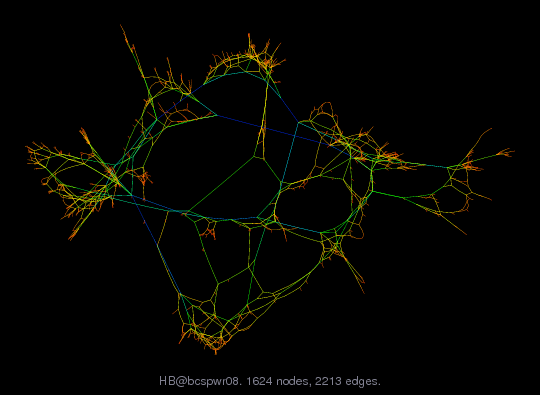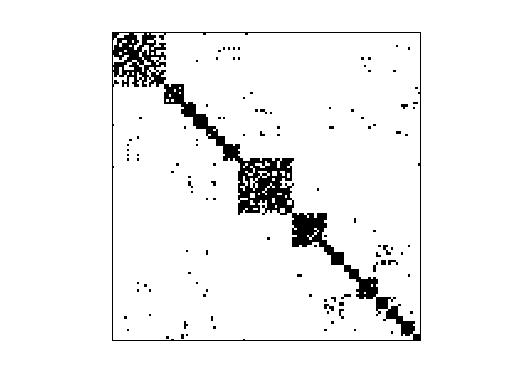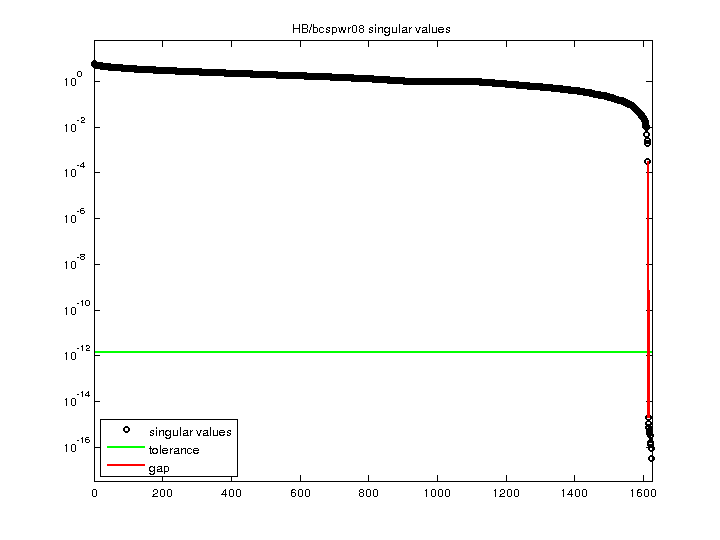Matrix: HB/bcspwr08

Description: SYMMETRIC STRUCTURE REPRESENTATION OF WESTERN US POWER NETWORK(undirected graph drawing)• Matrix group: HB
• download as a MATLAB mat-file, file size: 12 KB. Use UFget(20) or UFget('HB/bcspwr08') in MATLAB.

 Matrix properties number of rows 1,624 number of columns 1,624 nonzeros 6,050 structural full rank? yes structural rank 1,624 # of blocks from dmperm 1 # strongly connected comp. 1 explicit zero entries 0 nonzero pattern symmetry symmetric numeric value symmetry symmetric type binary structure symmetric Cholesky candidate? yes positive definite? no

 author B. Dembart, J. Lewis editor I. Duff, R. Grimes, J. Lewis date 1981 kind power network problem 2D/3D problem? no

 Ordering statistics: result nnz(chol(P*(A+A'+s*I)*P')) with AMD 5,623 Cholesky flop count 2.5e+04 nnz(L+U), no partial pivoting, with AMD 9,622 nnz(V) for QR, upper bound nnz(L) for LU, with COLAMD 8,544 nnz(R) for QR, upper bound nnz(U) for LU, with COLAMD 16,961

 SVD-based statistics: norm(A) 5.78591 min(svd(A)) 3.44732e-17 cond(A) 1.67838e+17 rank(A) 1,613 sprank(A)-rank(A) 11 null space dimension 11 full numerical rank? no singular value gap 1.54455e+11

 singular values (MAT file): click here SVD method used: s = svd (full (A)) ; status: ok